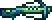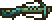•类型 \l!--\n --\g\{\{#vardefine:_typepage\!\{\{#or:\{\{l10n\!item_infobox\!typepage:@@@@\}\}\!\{\{tr\!\{\{ucfirst:@@@@\}\}\!link\ey\}\}\}\}\}\}\l!--\_\n --\g\{\{#vardefine:_typetext\!\{\{#or:\{\{l10n\!item_infobox\!type:@@@@\}\}\!\{\{tr\!\{\{ucfirst:@@@@\}\}\}\}\}\}\}\}\l!--\_\n --\g\{\{nowrap\!class\etag\!\{\{ifexists\!\{\{#var:_typepage\}\}\!\(\(\{\{#var:_typepage\}\}\!\{\{#var:_typetext\}\}\)\)\!\{\{#var:_typetext\}\}\}\}\}\}\l!--\n --\g 箭 36 (远程) 2（很弱击退力） 4% 22（快速度） 9.5180 需要 1 份

使用 \{\{#lstmap:\$soundfiles\$\!,\!%%%%\!\l!--\_print\_sounds\n --\g\lspan\_style\e"margin-left:2px;"\g\{\{sound\!\!\{\{trim\!%%%%\}\}\}\}\l/span\g\l!--\n --\g\!\}\}

!!Error: （请输入有效的值。参见Template:Modifier。）

• (10)

total: 1 row(s)